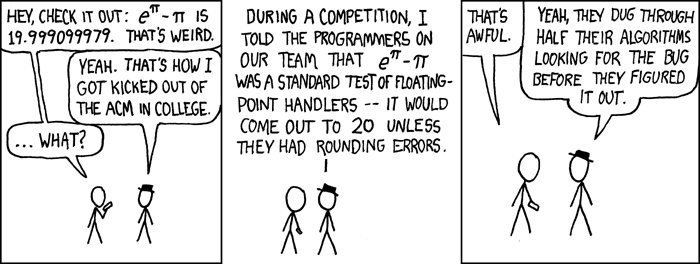# 217: e to the pi Minus pi

(Redirected from 217)
 e to the pi Minus piTitle text: Also, I hear the 4th root of (9^2 + 19^2/22) is pi.

## Explanation

e is a mathematical constant roughly equal to 2.71828182846. π is another, roughly equal to 3.14159265359. Both are transcendental numbers.

The first panel discusses eππ, which is around 19.999099979 — very close to 20. Black Hat explains how he tricked a programming team into believing that eππ really equals 20 - instead of just being weirdly close - thus that any noticeable deviation from 20 results from errors in the code. This made them waste a lot of time trying to find a nonexistent bug until they realized that Black Hat was lying (clearly they had not known him for very long, and clearly they weren't very knowledgeable in mathematics).

Floating point numbers are how computers store non-integer real numbers as decimals — or rather, in most cases, approximate them: infinite amounts of data would be required to represent most numbers in decimal form (exceptions are whole numbers and terminating decimals). The "floating-point handlers" would be the code performing the eππ calculation.

ACM is the Association for Computing Machinery, sponsoring the International Collegiate Programming Contest. It is likely that it was this competition, in which Black Hat wasted his teams time, for which he got kicked out.

Some random facts about the math here:

• eππ is an irrational number, but this is not a trivial fact. It was proven by Yuri Valentinovich Nesterenko in the late 20th century.
• The mysterious almost-equation is believed to be a mathematical coincidence, or a numerical relationship that "just happens" with no satisfactory explanation. It can be rearranged to (π + 20)i ≈ −1, so cos(ln(π + 20)) ≈ −1. Piling on a few more cosines gives cos(π cos(π cos(ln(π + 20)))) ≈ −1, which is off by less than 10−35!

The title text pokes fun at another coincidence: ∜(9² + 19²/22) ≈ 3.1415926525, close to π (deviating only in the 9th decimal place). The humor comes from the fact that π is transcendental. Transcendental numbers are numbers that cannot be expressed through basic arithmetic with integers; one cannot end up with the exact value for any transcendental number (including π) by adding, subtracting, multiplying, dividing, exponentiating, and/or taking the nth root of any rational number, meaning the title text cannot possibly be true.

A much later comic, 1047: Approximations, puts forth quite a few more mathematical coincidences.

## Transcript

Cueball: Hey, check it out: eπ − π is 19.999099979. That's weird.
Black Hat: Yeah. That's how I got kicked out of the ACM in college.
Cueball: ...what?
Black Hat: During a competition, I told the programmers on our team that eπ − π was a standard test of floating-point handlers -- it would come out to 20 unless they had rounding errors.
Cueball: That's awful.
Black Hat: Yeah, they dug through half their algorithms looking for the bug before they figured it out.add a comment! ⋅add a topic (use sparingly)! ⋅refresh comments!

# Discussion

Asserting that the programmers' algorithms truncated to three decimal digits is an unsupported and unnecessary extrapolation. Most floating-point implementations use binary, not decimal, and 19.999099979 looks very much like a rounding error in binary floating-point that has accumulated over several operations. Daddy (talk) 12:39, 29 April 2013 (UTC)

Fixed. Xhfz (talk) 22:57, 16 August 2013 (UTC)

The third bullet-point above needs changing... (9^2+(19^2/22))=97.4090909091 which is close to pi to the fourth power, so it should be (as noted in the text) (9^2+(19^2/22))^1/4 Squirreltape (talk) 19:27, 25 February 2014 (UTC)

Actually, in-case you didn't notice, it says "∜(9² + 19²/22)", not just the sum on its own. I checked the sum on my calculator, and it is equal to what the page is saying. "∜(9² + 19²/22)" means "4th root of (9^2+19^2/22)" (What the title text is saying), or on Windows Calculator, "(9^2+19^2/22) yroot(4)" (Basically what the sum is saying). So, the 3rd bullet point is correct. --Katavschi (talk) 22:48, 23 April 2014 (UTC)

It says above that (π + 20)^i ≈ -i, but this should be (π + 20)^i ≈ -1. Proof: π + 20 ≈ e^π => (π + 20)^i ≈ (e^π)^i = e^(πi) = -1.

The ACM competitions are famous for being under tight time pressure. Making your own team waste time would absolutely get you kicked out (and make enemies) Mountain Hikes (talk) 04:40, 23 September 2015 (UTC)

"If they thought about the mathematics"

hm, are you saying it is obvious that e^ pi - pi is not 20? How would you know without approximating it? The sum of two irrationals is not necessarily irrational. 162.158.34.194 01:58, 26 October 2015 (UTC)

approximate e^pi using slightly bigger numbers than e and pi (say e: 2.7183 and pi: 3.1416) and subtract a value that is slightly smaller than pi (say 3.1415). The result is less than 20 and a upper limit for e^pi - pi 141.101.93.49 19:59, 22 August 2016 (UTC)